### Home > MC2 > Chapter 8 > Lesson 8.1.2 > Problem8-25

8-25.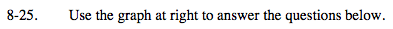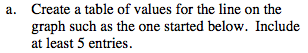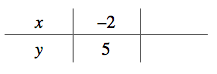Choose a few x-coordinates that can be seen in the graph. Estimate their y-coordinates.
Notice the scaling of the graph.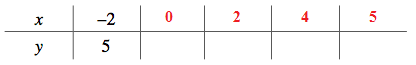2, −1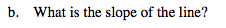$\text{Slope }= \frac{\text{rise}}{\text{run}}$

Notice that since the line slopes downward, its slope will have a negative value.

$-\frac{3}{2}$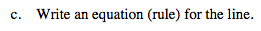Try looking for a pattern.

Notice that at x = 0 the y-coordinate is 2.
Try writing an equation, and then check your answerby substituting points found in (a).

$y = - \frac{3}{2} x + 2$